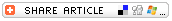Home >> A Tsl >> Archives >> 06 1 >> Perplexing Puzzles

#Perplexing Puzzles## Subjects

• Algebra
• Mathematics
-- Simple Graphing
-- Simple Statistics

• 3-5
• 6-8

## Brief Description

Perplexing Puzzles allows students to work in groups to put a puzzle together. Use this lesson to teaching graphing or the concept of a scatter plot.

## Objectives

Students
• understand the concept of a scatter plot. (Younger students will develop an understanding of simple graphs.)
• plot points for time and number of puzzle pieces put together.
• discuss or write about what their graphs show.

## Keywords

scatter plot, graph, x axis, y axis, puzzle, jigsaw puzzle

## Materials Needed

• jigsaw puzzles of about 250-500 pieces, one puzzle per group of 4 students; puzzles should be of same level of difficulty for all groups (very difficult puzzles are fine)
• stopwatch (optional)

## The Lesson

Arrange students into groups of 4. Provide each group with a jigsaw puzzle of similar difficulty. The puzzles might be between 250 and 500 pieces. Extra difficult puzzles are fine.

Give student groups about 5 minutes to organize their puzzle pieces. In this time, they should turn over all the pieces so the picture sides are up, and they can begin to separate outside-edge pieces from inner pieces.

Provide a class period for groups of students to work on putting together their puzzles. Set a stopwatch for 5-minute intervals. At each interval, ask students to record the number of puzzle pieces put together during the previous 5 minutes. (Note: Groups may not finish the entire puzzle in the class period, but that is fine.)

Allow students to work on the puzzle for a full class period. The next class period (or as homework), have students create graphs to show the number of puzzle pieces put together by the students in their group for each 5-minute interval.

• Young students might create bar graphs. Each bar will illustrate the number of pieces put together in a different 5-minute interval.
• Older students will create scatter-plot graphs. They will mark the x axis of their graphs "Time" and the y axis "Number of Puzzle Pieces Put Together" and plot the points on the graph.

## Assessment

Have students use their completed graphs to write an explanation of what the graphs show. In their writing students should explain the relationship between time and the number of puzzle pieces put together. They might answer questions such as

• Why does the graph look the way it does?
• What variables affected the number of pieces put together at different intervals?
• Based on the collected data, how much time do they estimate it would take the group to complete the entire puzzle?

Younger students might answer simpler questions such as

• What was your group's strategy for putting the pieces together?
• Was your group successful at completing the puzzle? Why or why not?
• Who was the "best" in your group at finding pieces that fit together?

As a culminating activity, students might complete their puzzles and put puzzle glue on them. Once dry, the puzzles could be attached to classroom ceiling tiles as decoration.

## Submitted By

Melissa Thomas, Dacula (Georgia) Middle School

Education WorldÂ®